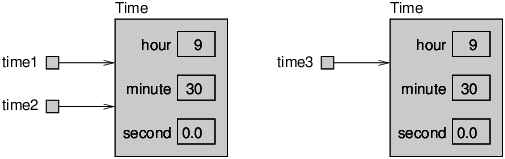Skip to main content

# 2.7: The equals Method

We have seen two ways to check whether values are equal: the == operator and the equals method. With objects you can use either one, but they are not the same.

• The == operator checks whether objects are identical; that is, whether they are the same object.
• The equals method checks whether they are equivalent; that is, whether they have the same value.

The definition of identity is always the same, so the == operator always does the same thing. But the definition of equivalence is different for different objects, so objects can define their own equals methods.

Consider the following variables:

Time time1 = new Time(9, 30, 0.0);
Time time2 = time1;
Time time3 = new Time(9, 30, 0.0);


Figure 11.7.1 is a state diagram that shows these variables and their values.Figure $$\PageIndex{1}$$: State diagram of three Time variables.

The assignment operator copies references, so time1 and time2 refer to the same object. Because they are identical, time1 == time2 is true.

But time1 and time3 refer to different objects. Because they are not identical, time1 == time3 is false.

By default, the equals method does the same thing as ==. For Time objects, that’s probably not what we want. For example, time1 and time3 represent the same time of day, so we should consider them equivalent.

We can provide an equals method that implements this notion of equivalence:

public boolean equals(Time that) {
return this.hour == that.hour
&& this.minute == that.minute
&& this.second == that.second;
}


equals is an instance method, so it uses this to refer to the current object and it doesn’t have the keyword static. We can invoke equals as follows:

time1.equals(time3);


Inside the equals method, this refers to the same object as time1, and that refers to the same object as time3. Since their instance variables are equal, the result is true.

Many objects use a similar notion of equivalence; that is, two objects are equivalent if their instance variables are equal. But other definitions are possible.